Mathematics

# Vectors and their Operations

258 views

Table of Contents

17 September 2020

Read time: 5 minutes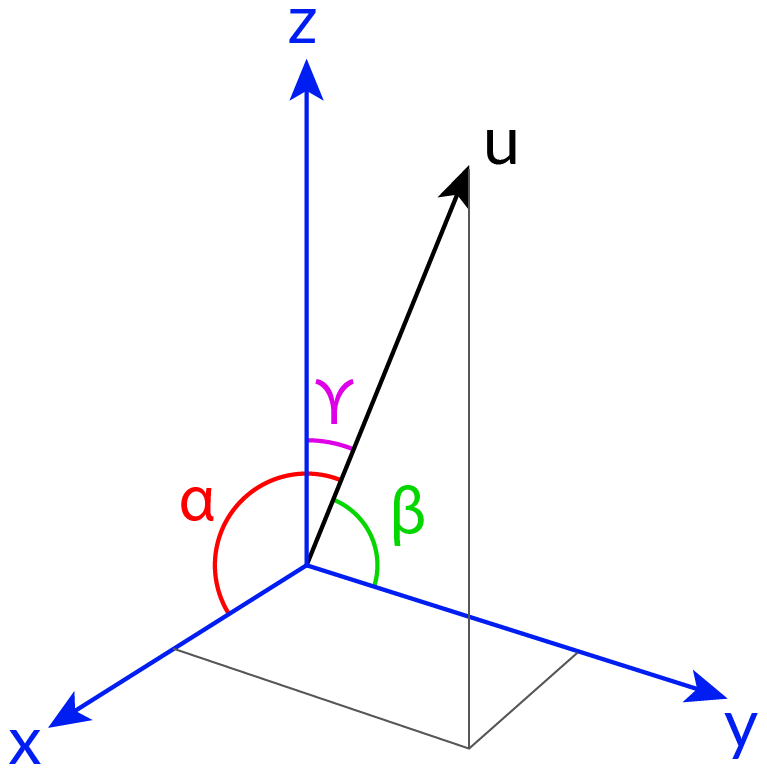What is a vector?

How are vectors used in mathematics?

A Vector has both magnitude and direction. Vectors are widely used to describe physical quantities. This blog will talk about performing different mathematical operations on vectors, finding the angle between two vectors with examples.

## Vectors and their Operations - PDF

If you ever want to read it again as many times as you want, here is a downloadable PDF to explore more.

 📥 Vectors and their Operations - PDF Download

Also read:

## Vector - Definition

• A vector stores two kinds of information, one is which direction it is pointing to and another one is how long it is as shown in the figure.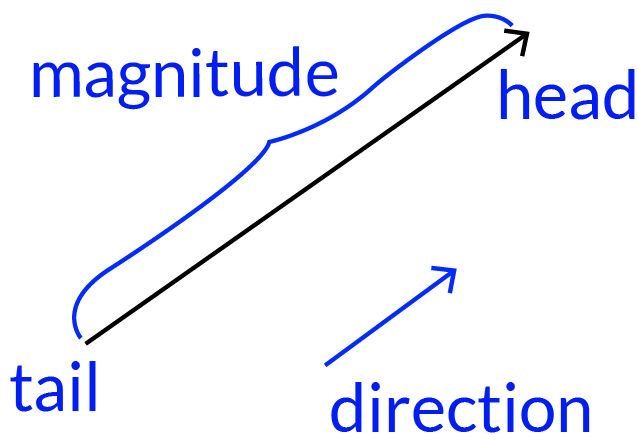Example: An example of a vector is displacement. Displacement tells us how far away we are from the fixed point and also in which direction is relative to that point. Another example of a vector is velocity. Velocity is nothing but the speed in a particular direction. The magnitude represents its length and an arrow indicates the direction. The pedaling of the pedal boat is a great example, it has the length and which direction it is pointed out.

• Vectors are indicated by the letter $$\rm{V}$$

• The magnitude of the vector is $$\rm{|V|}$$, which is a scalar.

• Two vectors with both the same magnitude and direction would be considered as the same vector.

• A vector with negligible length will be denoted as zero vector. So, a zero vector has no direction.

## How are vectors different from scalars?

Vector: Both Magnitude and Direction are present.

Scalar: Only the magnitude represented in a physical quantity is called a scalar. It is a number like $$3$$ or $$3.5$$ or $$-5.7$$

For example, $$\text{Kc}$$, the scalar $$\rm{K}$$ times the vector $$\rm{c}.$$

## How to find the magnitude of a vector?

The magnitude of a vector is shown as $$|\rm{v}|.$$ It is also known as the norm.

How to calculate the magnitude of a 2D vector? Having only x and y axes.

Using the Pythagorean theorem!

$$|\rm{v}| =\sqrt {{x^2} + {y^2}}$$

Example:

Vector is given as $$\rm{A} = (6, 8)$$, then apply the Pythagorean theorem, it's a square root of the sum of the square of the magnitude and the direction of the vector A.

\begin{align}|\text{A}|&=\sqrt{6^2+ 8^2}\\&=\sqrt{36+ 64}\\&=\sqrt{100}=10\end{align}

Unit vectors Whenever the magnitude of a vector has the value $$1$$, it is called a unit vector. To find the unit vector in the direction of a, you simply divide the vector by its magnitude.

$$\hat{\rm{u}}= \frac{a}{❘ a ❘}$$

## What are the different Vector Operations?

There are so many operations are performing on vectors are as follows:

• Addition among vectors
• Subtraction among vectors
• Multiplication of scalar with the vector
• The dot product
• The cross product

## Addition of two vectors (Adding Vectors)

When we are placing two vectors $$\rm{A}$$ and $$\rm{B}$$ end to end, the vector from the start of the first vector to the end of the second vector is their addition of vectors and it is represented as $$\rm{A + B}$$, a vector addition can add any number of vectors.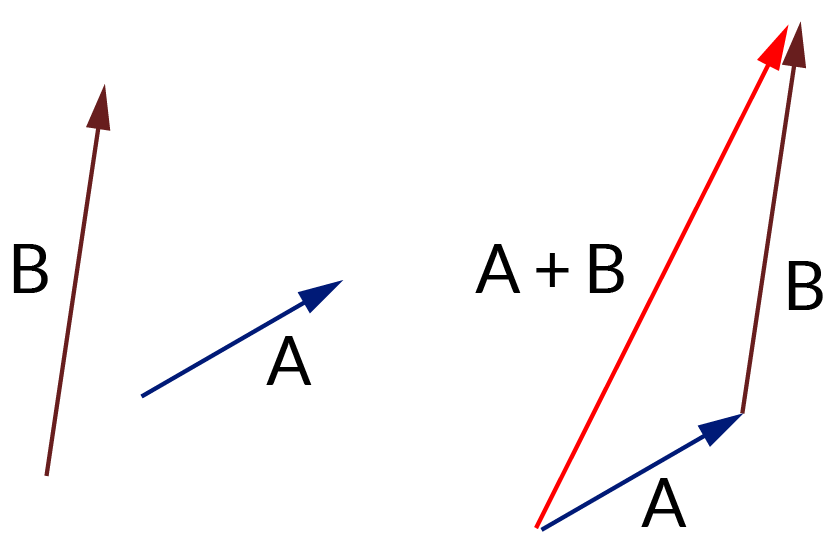### Example of Adding vectors:

$$\rm{a} = (12, 8)$$ and $$\rm{b}= (6, 25)$$  then $$\rm{C} = ( a_x+ b_x , a_y + b_y)$$

$$\rm{C}= (12+6 , 8+25)\\C=(18, 33)$$

Therefore the addition of vectors is $$\rm{C}= (18, 33).$$

## Laws of the addition of vectors:

### 1. The commutative law:

Adding vectors a and b is equal to its addition of vectors b and a.In both sides have the same answer. It is stated as, $$\rm{a + b = b + a}$$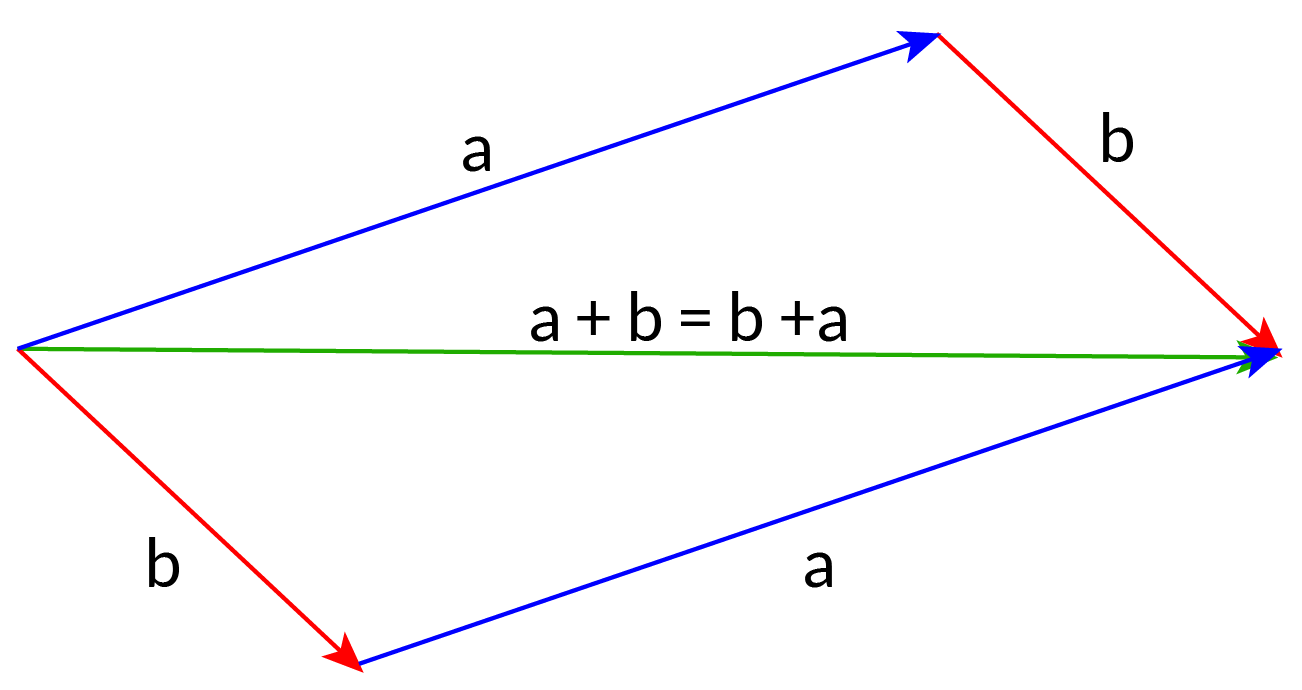This law is also known as the parallelogram law. The diagonal of the parallelogram is equal to its sum of their pair of two edges.

### 2. The associative law:

It is the addition of a and b then it is added to the vector c which is equal to the sum of the vectors b and c then added to the vector a. It gives the same result on both sides. It is stated as,

$$\rm{(a + b) + c = a + (b + c)}$$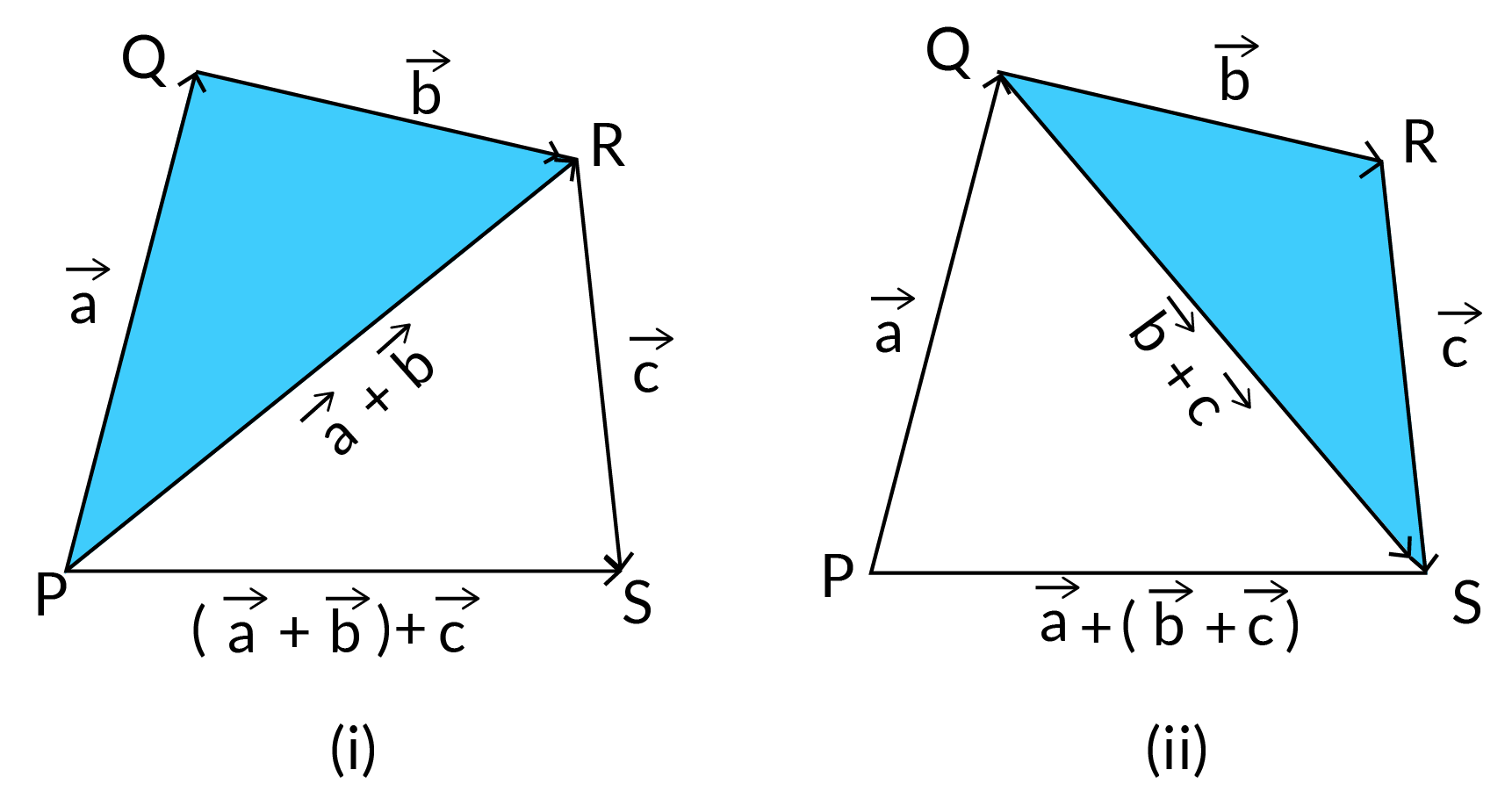The sum of the vectors a and b, then Added with c which is equal to the sum of b and c is added with a.

## Subtraction of two vectors (Subtracting Vectors)

The directed end of the two vectors is summed together to give the subtraction of the vectors. The negative sign of a vector indicates the opposite direction of the vector. Subtracting vectors is similar to adding vectors.

Example: - a is the opposite of -a, both have the same magnitude.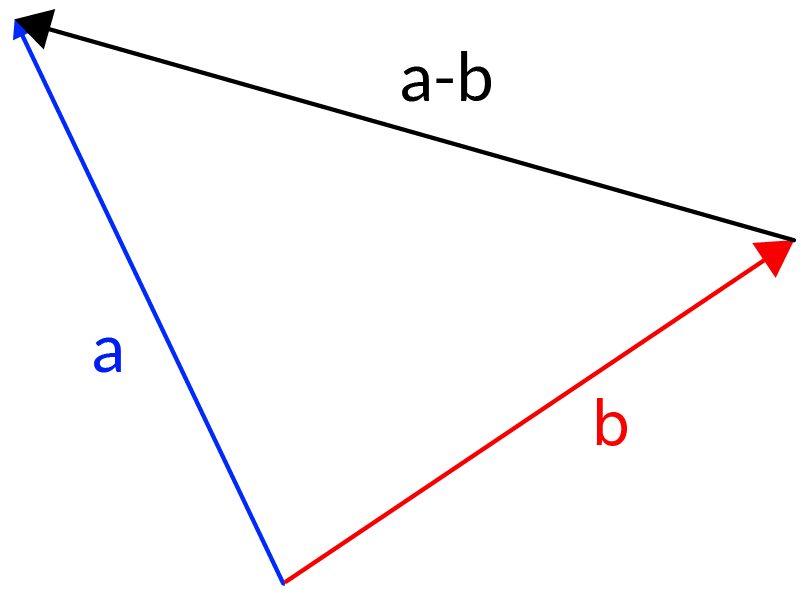When we are adding one vector b with the opposite sign of others must be like this $$\rm{b} + (-a) = b - a$$

Which gives a vector subtraction. As we know that $$\rm{a + x = b}$$, then $$\rm{b - a = x}$$, which is the scalar subtraction gives vector x.

### Example of Subtraction

Subtract $$\rm{k} = (5, 6)$$ from $$\rm{C}= (14, 3)$$

\begin{align}\rm{A}&= \rm{c} + -k\\\rm{A} &= (14, 3) + - (5, 6)\\&= (14, 3) + (-5, -6)\\A&= (9, -3)\end{align}

## How to multiply 2 vectors?

Vector multiplication is doing the product of two or more vectors with each other. It may be a Dot product or cross product.

### Dot product of Vectors:

It involves the multiplication of two vectors, giving a scalar quantity. It is also known as “Scalar product”. It is defined as the product of the magnitudes of the two vectors and the cosine of the angle between the two vectors.$$θ$$ applies in both 2D and 3D. Thus,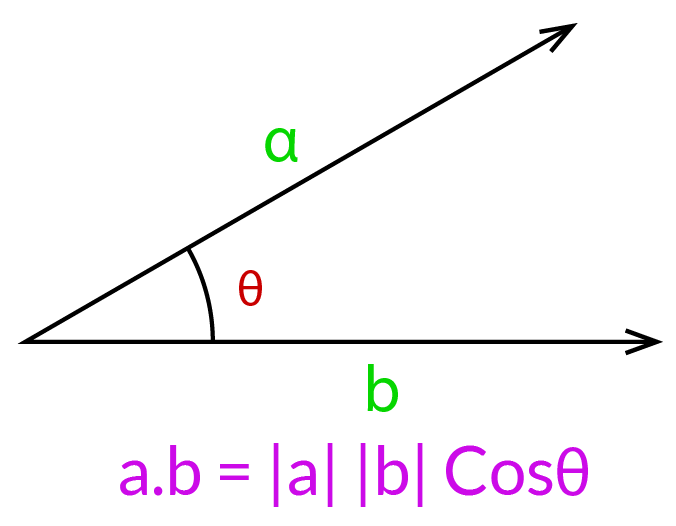$$\rm{A.B} = ||A|| ||B|| cos θ$$

Example of Dot product:

\begin{align}\rm{a.b} &= |a|× |b|× \text{Cos } θ\\&= 10 × 15 × \text{Cos } 60^{o}\\&= 10 \times 15 \times \frac{1}{2}\end{align}

One of the useful examples for the dot product is to calculate the geometric calculations of the mirror reflection from a surface. The dot product of orthogonal (perpendicular) vectors is zero, so a. $$\rm{b} = 0$$ then we know that the vectors must be orthogonal.

1. The dot product of two vectors is positive when the magnitude of the angle between is less than $$90^{\circ}$$.
2. The dot product of two vectors is negative when the magnitude of the angle is greater than $$90^{\circ}$$

### Cross product of Vectors:

The cross product of the two vectors is a vector that is perpendicular to its original vectors. A binary operation is involved in two vectors that give another vector. This is also called the “vector product”.

It is defined as the product of the magnitude of two vectors and the sine of the angle between the two vectors.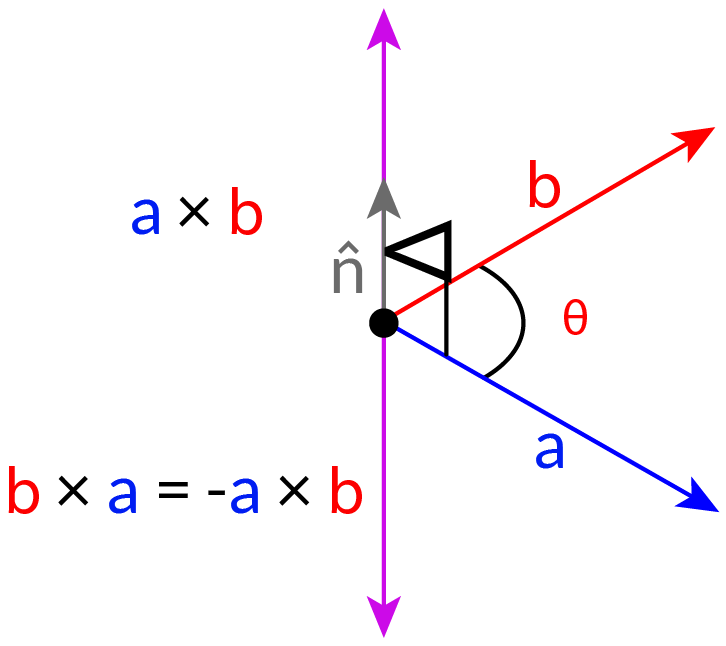$$\rm{A} \times B = ||A|| ||B|| \text{Sin θ}\, n$$

Where n is the unit perpendicular to the plane determined by the vectors A and B.

Example of Cross product :

Cross product of $$\text{a} = (3, 4, 5)$$ and $$\text{b}= (6, 7, 8)$$

\begin{align}\rm{}C_x &= \rm{}a_y b_z - a_z b_y = (4 × 8 - 5 × 7) = -3\\ \rm{}C_y &= \rm{}a_z b_x- a_x b_z = (5 × 6 - 3 × 8) = 6\\ \rm{}C_z &= \rm{}a_x b_y – a_y b_x = (3 × 7 – 4 × 6) = -3\\ \rm{}a × b& = (-3, 6, -3)\end{align}

Another example of the cross product is to calculate the area of a triangle. Suppose we have a triangle whose length is a and b and the angle between their sides is θ. The cross product is used to calculate the area. One more example for cross product is the right-hand rule. Before you take this rule, you must use your right hand, you spread your Index, middle, and thumb fingers perpendicular to each other. Index finger in the direction of the first vector. Middle finger in the direction of the second vector and the thumb points in the direction of the cross product.

## How to multiply a vector by a scalar?

A scalar is several times the vector will be added that is multiplied with the vector having both magnitude and direction. So the real number will be multiplied with both the values of the vectors.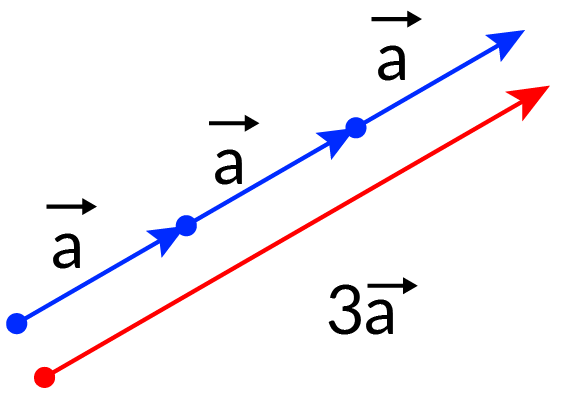Example: How to multiply the vector in 2 Dimension $$\text{V }(5,2)$$ by scalar $$4$$

$$\text{A} = 4v = (4 × 5, 4 × 2)\\\text{ A} = (20, 8)$$

The direction will remain the same here, but its magnitude is increased by four times.

## How to find the angle between two vectors?

Two vectors are separated by angle 𝛉. To calculate the angle between the vectors using the following formula. We know that the dot product of the vectors is given by, “$$\text{a .b} = |\text{a} | |\text{b} | \text{cosθ}$$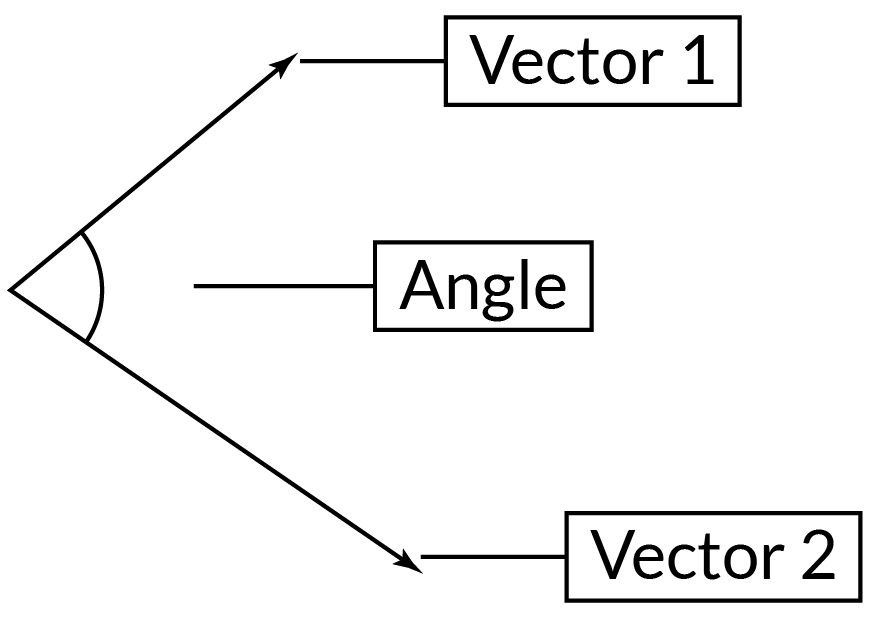Thus the angle between the two vectors is given as “$$\rm{θ = cos^{-1} a^{-1} .b^{-1} |a | |b|}$$

## How are Vectors used in geometry?

There is a useful theorem in geometry called the mid-point theorem. In this theorem, we take two points A and B, defined with respect to an origin O. Let us write a for the position vector of A, and b for the position vector of B. We can join A and B with a line, to give a triangle.

Now take the midpoint M of the line OA, and the mid-point N of the line OB, and join M to N with a line. Can we say anything about the relationship between the line MN and the line? We can answer this very easily with vectors. We can write the vector for the line segment AB as $$\text{AO + OB}$$. Now AO is the reverse of the vector a, so it is -a. And OB is the same as the vector b.

Now we can compare AB and MN. From our calculation, we can see that MN is $$\frac{1}{2}\text{AB}$$. So, as this is a vector equation, it tells us two things. First, it tells us about the magnitude, so that $$\text{MN} = \frac{1}{2}\text{AB.}$$ Also, it tells us that MN and AB must be in the same direction so that $$\text{MN//AB.}$$ This is called the mid-point theorem for a triangle. It states that if you join the mid-points of two sides of a triangle then the resulting line is equal to half of the third side of the triangle, and is parallel to it.

## Summary

Here, we discussed vectors, the magnitude of vectors, operations like Addition, Subtraction, Dot Product, and Cross Product of Vectors. We also covered the angle between two Vectors with suitable examples.

Learning about vectors requires consistent practice and a complete grasp of the concept. Practice problems regularly to master the concept. Learn mathematics with us, sign-up for a free trial

Written by Suganya Balaji, Cuemath Teacher

## About Cuemath

Cuemath, a student-friendly mathematics and coding platform, conducts regular Online Live Classes for academics and skill-development, and their Mental Math App, on both iOS and Android, is a one-stop solution for kids to develop multiple skills. Understand the Cuemath Fee structure and sign up for a free trial.

## What is the difference between scalar and vector? (Scalar vs Vector)

 Vector Scalars Vectors have magnitude as well as the direction. Scalars have magnitude only.

## What are orthogonal vectors?

Orthogonal vectors are perpendicular to each other i.e. the dot product of the two vectors is zero.

## What are the orthonormal vectors?

A set of vectors S is orthonormal if every vector in S has magnitude 1 and the set of vectors are mutually orthogonal.

## What are linearly independent vectors?

An orthogonal set of non-zero vectors is linearly independent.

## What are unit vectors?

A unit vector has a magnitude (length) is one.

## What are parallel vectors?

Parallel vectors have the same direction angles but may have different magnitudes.

## What are perpendicular vectors? (How do we know $$2$$ vectors are perpendicular?)

The dot product of two perpendicular vectors is zero.

## External References

Vectors in Computer Science

GIVE YOUR CHILD THE CUEMATH EDGE
Access Personalised Math learning through interactive worksheets, gamified concepts and grade-wise courses
Learn More About Cuemath